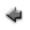previous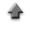home

# Constrained 2 dimensional function model fitting

You can fit an arbitrary constrained function model f(x,y)=0 to a data set including errors in both the abscissa(x) and the ordinate(y) data. To start, click "2D Constrained Fit" tab on the application.
If you use the sample data, click here, and save it in the appropriate directory (or folder).

When you fit a function (in this case, a Lorentzian function) to the sample data,

1. Click "Open data file", and specify the data file prepared according to the above instruction.
2. When clicking the "Plot" button bellow, the plot is displayed.
3. When clicking the "Fit" button in the plot window, a result of the fitting is displayed.

## Data

1. Each label for data can be set in the first row in the data file. The label can not include spaces. Each label has to be delimited by space or tab.
2. Data must be inputted by normal width.
3. Data must be inputted in the data file corresponding to the following combinations of the data type for x and y.
• When the data type is "Gaussian-Gaussian" combination (x and y follow Gaussian distributions, respectively), the data must be inputted in order of x, the error of x (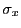), y and the error of y (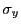) which are delimited by space or tab. Change the line, and input the data of the following row similarly until the input of the last data ends.
 x1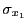y1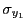x2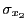y2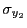. . . . . . . . . . . . xn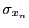yn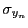• When the data type is "Gaussian-Poisson" combination (x follow Gaussian distributions and y Poisson distributions), the data must be inputted in order of x, the error of x () and y which are delimited by space or tab. The errors of y (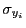) correspond to the square root of the values of y (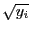). Change the line, and input the data of the following row similarly until the input of the last data ends.
 x1y1 x2y2 . . . . . . . . . xnyn
• When the data type is "Poisson-Gaussian" combination (x follow Poisson distributions and y Gaussian distributions), the data must be inputted in order of x, y and the error of y () which are delimited by space or tab. The errors of x (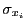) correspond to the square root of the values of x (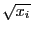). Change the line, and input the data of the following row similarly until the input of the last data ends.
 x1 y1x2 y2. . . . . . . . . xn yn• When the data type is "Poisson-Poisson" combination (both x and y follow Poisson distributions, respectively), the data must be inputted in order of x and y which are delimited by space or tab. The errors of x () correspond to the square root of the values of x (). The errors of y () correspond to the square root of the values of y (). Change the line, and input the data of the following row similarly until the input of the last data ends.
 x1 y1 x2 y2 . . . . . . xn yn
4. In addition, you can use the table data form in the data file. You may include the column data in the data file which are not used to fit. In that case, the specification of the columns in the data file used to fit is needed at the line "Data columns" in the following "Input" instruction.

## Inputs

1. Click "Open data file", and specify the data file prepared according to the above instruction.
2. When the labels for data are used, check the "Data labels".
3. The columns in the data file to be used to fit a function model can be specified at the line "Data columns". For instance, in the case that the data type is "Gaussian-Gaussian" combination, when x are put in the third column, the error of x in the forth column, y in the fifth column and the error of y in the sixth column in the data file, specify "3 4 5 6", and check the "Data columns" box.
4. The range for x axis in the plot can be specified at the line "Data range". In that case, specify the range for x axis (the numerical values must be delimited by space), and check the "x range" box. For instance, when you want to assume the range for x to be 0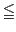x15, specify "0 15", and check the "x range" box.
5. The range for y axis in the plot can be specified at the line "Data range". In that case, specify the range for y axis (the numerical values must be delimited by space), and check the "y range" box. For instance, when you want to assume the range for x to be 0y15, specify "0 15", and check the "y range" box.
6. Select the data type at the line "Data type" according to the combination of the x and y data type.
7. The title of the graph can be specified at the line "Graph title". Click the "Set" button after describing it.
8. The label of the abscissa in the graph can be specified at the line "Label of abscissa". Click the "Set" button after describing it.
9. The label of the ordinate in the graph can be specified at the line "Label of ordinate". Click the "Set" button after describing it.
10. The explanatory note (legend) of the data can be specified at the line "Legend of data". Click the "Set" button after describing it.

## Outputs

1. The plot can be displayed when the "Plot" button is clicked.
2. A summary of the plot can also be displayed when the "Show data summary" button is clicked.

## Fitting a function to data

1. Input a function model at the line "Function f(x,y)", and click the "Set" button. The input of the function must follow the form of f(x,y)=0. About the input of the function, refer here.
2. This fitting algorithm requires the specification of initial values of the parameters included in the function model. Then, you must input the initial values of the parameters at the line "Parameters" which must be delimited by space, and click the "Set" button.
3. The range for x data to be fitted can be specified at the line "x data range". In that case, specify the range for x axis (the numerical values must be delimited by space), and check the "x data range" box. For instance, when you want to assume the range for x to be 0x15, specify "0 15", and check the "x data range" box.
4. Like the range for x data, the range for y data to be fitted can be specified at the line "y data range". In that case, specify the range for y in the plot (the minimum and the maximum values must be delimited by space), and check the "y data range". For instance, when you want to assume the range for y in the histogram to be 0y15, specify "0 15", and check the "y data range".
5. The explanatory note (legend) of the function can be specified at the line "Legend". Click the "Set" button after describing it.
6. When you use the second order derivatives of the function with respect to the parameters in computation of the parameter's errors, check the "2nd order derivatives" box.
7. A result of fitting can be displayed, when the "Fit" button is clicked, and the fitting function is also displayed on the graph.
8. When the "Show error matrix" button is clicked, the elements of the error matrix is displayed.
9. When the "Show residual graph" button is clicked, the plot of the residuals is displayed in another window.
10. When the "Show residuals" button is clicked, the estimations of x and y, and their residuals are displayed.

### Drawing a function represented by a parametric variable

You can draw a parametric function in the plot. About the function represented by parametric variables, refer here.
1. Input the parametric variable function for x at the line "parametric x", and click the "Set" button. Where the parameter name is x, and the constant name is p0 and p1, and so on.
2. If the constants (p0, p1, etc) are included in the parametric variable function of x, input the values delimiting by space at the "constants for x", and click the "Set" button.
3. Input the parametric variable function for y at the line "parametric y", and click the "Set" button. Where the parameter name is x, and the constant name is p0 and p1, and so on.
4. If the constants (p0, p1, etc) are included in the parametric variable function of y, input the values delimiting by space at the "constants for y", and click the "Set" button.
5. Specify the range for the parameter(parametric variable) x included in both the parametric variable functions of x and y at the "range of parameter" which the values must be delimited by space, and click the "Set" button.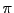(=3.14159...) can be specified as a unit other than the numerical value. For example, it can be specified as "2*pi", "1.5*pi", and so on.
6. Click the "Draw parametric function" button.

Kazushi Neichi